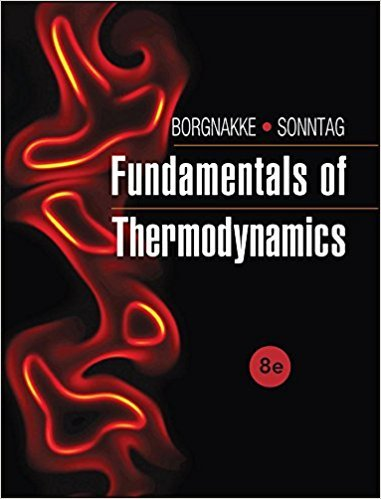×
Get Full Access to Fundamentals Of Thermodynamcs - 8 Edition - Chapter 6 - Problem 151hp
Get Full Access to Fundamentals Of Thermodynamcs - 8 Edition - Chapter 6 - Problem 151hp

×

# A rigid container with volume 200 L is divided into twoISBN: 9781118131992 58

## Solution for problem 151HP Chapter 6

Fundamentals of Thermodynamcs | 8th Edition

• Textbook Solutions
• 2901 Step-by-step solutions solved by professors and subject experts
• Get 24/7 help from StudySoup virtual teaching assistantsFundamentals of Thermodynamcs | 8th Edition

4 5 1 258 Reviews
14
3
Problem 151HP

Problem 151HP

A rigid container with volume 200 L is divided into two equal volumes by a partition, shown in Fig. P6.151. Both sides contain nitrogen; one side is at 2 MPa, 200°C and the other at 200 kPa, 100°C. The partition ruptures, and the nitrogen comes to a uniform state at 70°C. Assume the temperature of the surroundings is 20°C. Determine the work done and the net entropy change for the process.

FIGURE P6.151Step-by-Step Solution:
Step 1 of 3

GEOL 207 Environmental Geology Atmosphere - Nitrogen (N2) = 78% - Oxygen (O2) = 21% o Photosynthesis - Argon (Ar) = 0.9% o Stable and do not want to react - Carbon Dioxide (CO2) = 0.039% o Goes up and down. Has recently been going up - Water (H2O) = 10^-5% -- > 4% Nitrogen / Oxygen / and Argon Do NOT have a GRENHOUSE EFFECT Carbon Dioxide and Water DO have a GREENHOUSE EFFECT - Methane (CH4) o Smallest of our natural gases o Naturally occurring (soils) - Nitrous Oxide (N2O) o - Ozone (Ozone) o - Freons (CFCl) (Chlorofluorocarbons) o Methane, N2O, Ozone, Chlorofluorocarbons are measured in (ppm)! - Air Pressure o 1 atm o 1023 millibars

Step 2 of 3

Step 3 of 3

##### ISBN: 9781118131992

Since the solution to 151HP from 6 chapter was answered, more than 422 students have viewed the full step-by-step answer. This textbook survival guide was created for the textbook: Fundamentals of Thermodynamcs , edition: 8. The answer to “A rigid container with volume 200 L is divided into two equal volumes by a partition, shown in Fig. P6.151. Both sides contain nitrogen; one side is at 2 MPa, 200°C and the other at 200 kPa, 100°C. The partition ruptures, and the nitrogen comes to a uniform state at 70°C. Assume the temperature of the surroundings is 20°C. Determine the work done and the net entropy change for the process.FIGURE P6.151” is broken down into a number of easy to follow steps, and 72 words. This full solution covers the following key subjects: nitrogen, partition, assume, comes, contain. This expansive textbook survival guide covers 7 chapters, and 1462 solutions. Fundamentals of Thermodynamcs was written by and is associated to the ISBN: 9781118131992. The full step-by-step solution to problem: 151HP from chapter: 6 was answered by , our top Engineering and Tech solution expert on 08/03/17, 05:05AM.

Unlock Textbook Solution